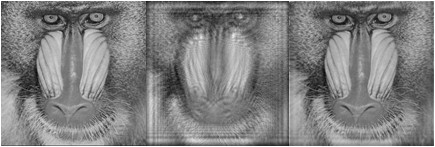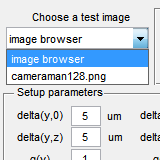# Inverse wave field imagingForward/backward modelingForward/backward modelingMulti-plane phase retrievalSparse reconstruction Publications Contacts

A wave field reconstruction from intensity/phase measurements is one of important problems in digital wave field imaging and holography. We are reconstructing the whole 3D object representation. Here we present a demo realizing a discrete-to-discrete model of the forward/backward wave field propagation considered in . The forward propagation is performed with the recent Discrete Diffraction Transform in the matrix form (M-DDT). The object reconstruction (backward diffraction propagation) by the Tikhonov regularized inverse of M-DDT is compared with the results obtained using different other methods (see Section "Comparing with..." in the GUI below):
• by truncated Singular Value Decomposition (trunc. SVD);
• by Discrete Diffraction Transform in the frequency domain with Recursive Regularized Inverse algorithm (FDDT RRI);
• by the conventional convolution algorithm (conv) of the single and double size;
• by the conventional angular spectrum decomposition (ASD) of the single and double size.
The whole forward/backward diffraction propagation modeling can be done with the proposed m-file demo_wrec.m : modeling parameters can be set manually or with the additional GUI, illustrated in Fig. 1.1. The object reconstruction using the Tikhonov regularized inverse of M-DDT is performed by default with the regularization parameter denoted in GUI by "reg. MDDT" (by "alpha" in the MATLAB file). Note, that in contrast to M-DDT, we present another regularization parameter for FDDT RRI: "reg. FDDT" in GUI or "beta" in demo_wrec.m The program demo_wrec works with gray-scale images only (a color image will be converted to a gray-scale one automatically).

The LASIP routines are available free-of-charge for educational and non-profit scientific research, enabling others researchers to understand and reproduce our work. Any unauthorized use of the LASIP routines for industrial or profit-oriented activities is expressively prohibited. Please read the LASIP limited licensebefore you proceed with downloading the files.

 CLICK HERE TO DOWNLOAD .ZIP PACKAGEWave field reconstruction

Using the presented demo you can test the quality of the wave field reconstruction for different setting parameters obtained by various algorithms. The M-DDT algorithm with the averaging ( “averaged matrices”) demonstrates aliasing free reconstructions. Numerically and visually the regularized inverse of M-DDT shows better performance comparing with the conventional techniques, and (in some cases) even better than the results computed with F-DDT RRI .Fig. 1.1. GUI for setup parameters setting (click to enlarge)

In order to make the setting of modeling parameters friendly, the graphical user menu has been developed (see Fig. 1.1).
You may select a test image from a list of *.png, *jpg and *.bmp images contained in the current directory with a pop-up menu (choose "image browser" to select a file from another directory), and set the type of modulation (amplitude or phase) in Section "Object type".

In Section "Setup parameters" you may specify the used wavelength lambda ("lambda"), pixel sizes at the object Delta_{y,0} xx Delta_{x,0} ("delta(y,0)" and "delta(x,0)") and sensor planes Delta_{y,z} xx Delta_{x,z} ("delta(y,z)" and "delta(x,z)"), and the image size ratio of the sensor planes with respect to the object plane (sensor redundancy) in the y- ("q(y)") and x- ("q(x)") directions, respectively. Using the additional button (!) all these settings can be saved in a file or loaded for a file.

In the section "Usage of averaging" you can choose whether you want to use so-called “averaged matrices” for the forward or/and backward propagations.
Computation of the averaged matrices is the most demanding element of the computational complexity of M-DDTjjj. These matrices can be calculated in advance for a set of parameters, saved and used for processing and modeling (see the check box "use saved data"). If you have already a number of saved matrices you can choose an appropriate one (see the dialogs for saving, using of existing files or loading (from a directory).

In Section "Propagation distances" the distances for the forward and backward propagations can be set in mm or with a factor of the "in-focus" distance dF. If "use 'in-focus' distance" is unchecked, both these distances can be modified. Otherwise these distances are the same and equal to the in-focus distance. By default (forward and backward propagations with averaging), the in-focus distance is calculated with respect to the minimum value of the image size ratio q (for more details see ).

In Section "Comparing with..." you can select alternative wave field reconstruction methods to compare their results with M-DDT.
Use the calculate button in order to perform the simulation of the forward/backward propagation for chosen setup settings. If saved matrices are used and current setup settings are different from those that were made for the saved data, then you will be prompted to confirm the use of the data. Clicking on the "Show me the differences" button the differences will be shown.

## References and publicationsV. Katkovnik, A.Migukin, J. Astola, “Backward discrete wavefield propagation modeling as an inverse problem: toward perfect reconstruction of wavefield distribution”, Appl. Opt. 48, 3407-3423 (2009).V. Katkovnik, J. Astola, and K. Egiazarian, “Discrete diffraction transform for propagation, reconstruction, and design of wavefield distributions”, Appl. Opt. 47, 3481-3493 (2008).V. Katkovnik, A.Migukin, J. Astola, “Digital 2D wavefield reconstruction based on novel two-matrix forward/backward propagation modeling”, ISMTII’2009, St. Petersburg, Russia, 2009.V. Katkovnik, A.Migukin, J. Astola, “Computational inverse holographic imaging: toward perfect reconstruction of wavefield distributions”, Fringe’09, Nuertingen, Germany, 2009.V. Katkovnik, A.Migukin, J. Astola, “Computational inverse coherent wave field imaging”, IEEE International Conference on Image Processing – ICIP’2009, Cairo, Egypt, 2009.